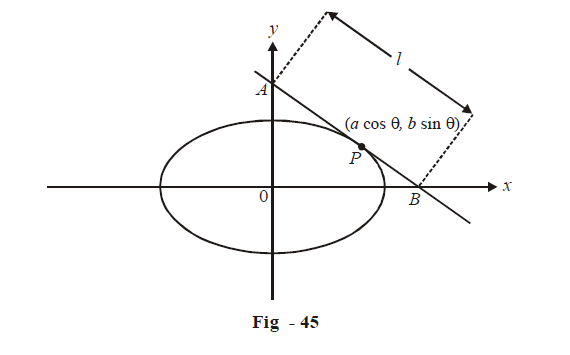# Applications of Derivatives Set 4

Go back to  'SOLVED EXAMPLES'

Example - 8

For what values of the parameter $$a$$ does the function$$f\left( x \right) = {x^3} + 3\left( {a - 7} \right){x^2} + 3\left( {{a^2} - 9} \right)x - 1$$ have a positive point of maximum?

Solution:    $$f'\left( x \right) = 3{x^2} + 6\left( {a - 7} \right)x + 3\left( {{a^2} - 9} \right)$$

For                  $$f\left( x \right)$$to have a maximum at some point

$$f'\left( x \right) = 0\,\,{\text{and}}\,f\left( x \right) < 0$$ for that point

\begin{align}&Now\,\,\,\,\,\,\,\,\,\,\,\,\,\,\,\,f'\left( x \right) = 0\\\\&\,\,\,\,\,\,\,\,\,\,\,\,\,\,\,\,\,\,\,\,\,\,\, \Rightarrow\quad 3{x^2} + 6\left( {a - 7} \right)x + 3\left( {{a^2} - 9} \right) = 0\\\\&\,\,\,\,\,\,\,\,\,\,\,\,\,\,\,\,\,\,\,\,\,\, \Rightarrow \quad {x^2} + 2\left( {a - 7} \right)x + \left( {{a^2} - 9} \right) = 0\\\\&\,\,\,\,\,\,\,\,\,\,\,\,\,\,\,\,\,\,\,\,\,\, \Rightarrow \quad x = - \left( {a - 7} \right) \pm \sqrt {58 - 14a} \,\,\,\,\,\,\,\,\,\,\,\,\,\,\,\,\,\,\,\,\,\,{\rm{ }}...{\rm{ (i) }}\end{align}

For $$f'\left( x \right)$$ to have real roots,

$$58\text{ }-\text{ }14a~>\text{ }0$$

\begin{align}\Rightarrow \quad a < \frac{{29}}{7}\,\,\,\,\,\,\,\,\,\,\,\,\,\,\,\,\,\,\,\,\,\,\,\,\,\,\,\,\,\,\,\,\,\,\,\,\,\,\,\,\,\,\,\,\,\,...{\rm{ }}\left( {ii} \right)\end{align}

Now we determine which of the roots of $$f'\left( x \right)$$ in (i) will give a local maximum and which will give a local minimum.

\begin{align}&\,\,\,\,\,\,\,\,\,\,\,\,\,\,\,\,\,\,\,\,\,\,f\left( x \right) = 6x + 6\left( {a - 7} \right)\\\\&\,\,\,\,\,\,\,\,\,\,\,\,\,\,\,\,\,\,\,\,\,\,\,\,\,\,\,\,\,\,\,\,\,\,\,\,\,\,\,\,\,\,\,\,\,\,\,\, = 6\left( {x + a - 7} \right)\\\\&\text{At}\,\,\,\,\,\,\,\,\,\,\,\,\,\,\,\,{x_1} = - \left( {a - 7} \right) + \sqrt {58 - 14a}\quad \Rightarrow\quad f''\left( x \right) = 6\sqrt {58 - 14a} > 0\\\\&\text {At}\,\,\,\,\,\,\,\,\,\,\,\,\,\,\,\,{x_2} = - \left( {a - 7} \right) - \sqrt {58 - 14a} \quad \Rightarrow\quad f''\left( x \right) = - 6\sqrt {58 - 14a} < 0\end{align}

Therefore, x = x2 is a point of local maximum. Since we want this to be positive.

\begin{align}&\qquad - \left( {a - 7} \right) - \sqrt {58 - 14a} > 0\\\\ &\Rightarrow \quad \,7 - a > \sqrt {58 - 14a} \end{align}

Upon squaring, we get

\begin{align}&\qquad\quad {a^2} - 9 > 0\\\\ &\Rightarrow\quad a < - 3\,\,{\rm{or}}\,\,a > 3\,\,\,\,\,\,\,\,\,\,\,\,\,\,\,\,\,\,\,\,\,\,\,\,\,\,\,\,\,...{\rm{ }}\left( {iii} \right)\end{align}

From (ii) and (iii),

$a < - 3\,\,\,\,{\rm{or}}\,\,\,3 < a < \frac{{29}}{7}$

Example - 9

Prove that the minimum intercept made by the axes on any tangent to the ellipse \begin{align}\frac{{{x^2}}}{{{a^2}}} + \frac{{{y^2}}}{{{b^2}}} = 1\end{align} is (a + b). Also, find the ratio in which the point of contact divides the intercept.

Solution: We can assume a variable point on the ellipse as$$P\left( {a\cos \theta ,b\sin \theta } \right).$$ We will first write down the equation of the tangent to the ellipse at this point and then find the length of the intercept of tangent between the axes in terms of $$\theta$$. The length can then be minimized with respect to $$\theta$$.Differentiating the equation of the ellipse, we get:

\begin{align}&\qquad\quad\frac{{2x}}{{{a^2}}} + \frac{{2y}}{{{b^2}}}\frac{{dy}}{{dx}} = 0\\\\& \Rightarrow \quad \frac{{dy}}{{dx}} = - \frac{x}{y} \cdot \frac{{{b^2}}}{{{a^2}}}\\\\ &\Rightarrow\quad {\left. {\frac{{dy}}{{dx}}} \right|_{\left( {a\cos \theta ,b\sin \theta } \right)}} = \frac{{ - b}}{a}\cot \theta \end{align}

Equation of tangent:   \begin{align}y - b\sin \theta = \frac{{ - b}}{a}\cot \theta \left( {x - a\cos \theta } \right)\end{align}

$$\Rightarrow \quad bx\cos \theta + ay\sin \theta = ab$$

x–intercept:    Put y = 0

$$\Rightarrow \qquad x=a\, \sec \theta$$

y-intercept:      Put x = 0

$$\Rightarrow \qquad y=b\text{ cosec }\theta$$

Length of tangent intercepted by the axes:

${l^2} = {a^2}{\sec ^2}\theta + {b^2} cosec^2$

For minimum

\begin{align}l,\frac{{d\left( {{l^2}} \right)}}{{d\theta }} = 0\,\,{\rm{and}}\,\,\frac{{{d^2}\left( {{l^2}} \right)}}{{d{\theta ^2}}} > 0\end{align}

\begin{align}\frac{{d\left( {{l^2}} \right)}}{{d\theta }} = 2{a^2}{\sec ^2}\theta \tan \theta - 2{b^2}cosec^2\theta \cot \theta \end{align}

This is 0 when:

\begin{align}&\quad\quad\;\;2{a^2}{\sec ^2}\theta \tan \theta = 2{b^2} cosec^2\theta \cot \theta \\&\Rightarrow \quad {\tan ^4}\theta = \frac{{{b^2}}}{{{a^2}}}\\& \Rightarrow\quad \tan \theta = \pm \sqrt {\frac{b}{a}} \end{align}

Verify that \begin{align}{\left. {\frac{{{d^2}\left( {{l^2}} \right)}}{{d{\theta ^2}}}} \right|_{\theta = {{\tan }^{ - 1}}\left( { \pm \sqrt {\frac{b}{a}} } \right)}} > 0\end{align}  (In fact$$\frac{{{d^2}\left( {{l^2}} \right)}}{{d{\theta ^2}}}$$, is always positive)

For minimum intercept:              $$\qquad \qquad\qquad\qquad l_{\min }^2 = {a^2}{\sec ^2}\theta + {b^2} cosec^2 \theta \left| {_{\tan \theta = \pm \sqrt {\frac{b}{a}} }} \right.$$

\begin{align}&{\left. { = \left\{ {{a^2}\left( {1 + {{\tan }^2}\theta } \right) + {b^2}\left( {1 + {{\cot }^2}\theta } \right)} \right\}} \right|_{\tan \theta = \pm \sqrt {\frac{b}{a}} }}\\\\ &= {a^2} + {b^2} + 2ab\\&= {(a + b)^2}\\&\Rightarrow \quad {l_{\min }} = \left( {a + b} \right)\end{align}

Referring to Fig - 45 for minimum intercept:

\begin{align} &\qquad \;P{Q^2} = {\left( {a\cos \theta - a\sec \theta } \right)^2} + {\left( {b\sin \theta } \right)^2} \\\\ &\qquad\qquad = {a^2}\frac{{{{\sin }^4}\theta }}{{{{\cos }^2}\theta }} + {b^2}{\sin ^2}\theta \\\\ &\qquad\qquad = {\sin ^2}\theta \left( {{a^2}{{\tan }^2}\theta + {b^2}} \right) \\\\ &\qquad\qquad = \frac{b}{{a + b}}\left( {{a^2} \times \frac{b}{a} + {b^2}} \right)\,\,\,\,\,\,\,\,\,\,\,\,\,\left( { \because \tan \theta = \pm \sqrt {\frac{b}{a}} } \right) \\\\ & \qquad\qquad= {b^2} \\\\&\Rightarrow\quad PQ = b\\\\& \Rightarrow\quad PR = a\,\,\,\,\,\,\,\,\,\,\,\,\,\,\,\,\,\,\,\,\,\,\,\left( {since\;QR = a + b} \right)\\\\& \Rightarrow \quad \frac{{PQ}}{{PR}} = \frac{b}{a} \end{align}

The minimum length intercept is divided in the ratio $$b:a$$ at the point of contact.

Learn from the best math teachers and top your exams

• Live one on one classroom and doubt clearing
• Practice worksheets in and after class for conceptual clarity
• Personalized curriculum to keep up with school